# DAV Class 5 Maths Chapter 4 Worksheet 4 Solutions

The DAV Class 5 Maths Book Solutions Pdf and DAV Class 5 Maths Chapter 4 Worksheet 4 Solutions of Fractional Numbers offer comprehensive answers to textbook questions.

## DAV Class 5 Maths Ch 4 Worksheet 4 Solutions

Question 1.
(a) $$\frac{4}{7}+\frac{11}{14}$$
Solution: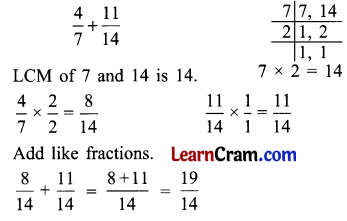(b) $$\frac{5}{8}+\frac{1}{6}$$
Solution:(c) $$\frac{1}{4}+\frac{2}{5}+\frac{7}{10}$$
Solution:(d) $$\frac{5}{2}+\frac{1}{8}+\frac{3}{4}$$
Solution:(e) $$\frac{2}{3}+\frac{1}{10}+\frac{7}{5}$$
Solution: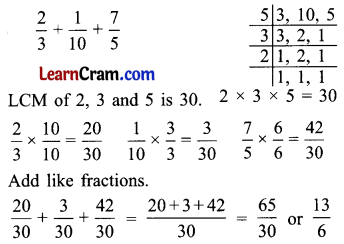(f) $$\frac{1}{3}+\frac{12}{33}+\frac{2}{11}$$
Solution: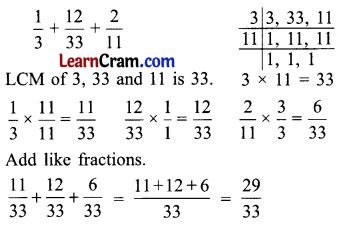(g) $$2+4 \frac{1}{3}+\frac{7}{5}$$
Solution:(h) $$4 \frac{1}{6}+2 \frac{2}{5}+1 \frac{1}{3}$$
Solution: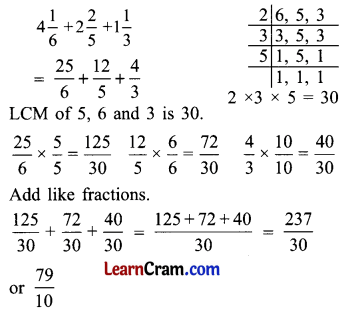(i) $$7+\frac{9}{10}+\frac{2}{3}$$
Solution: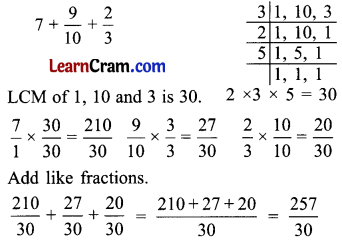Question 2.
Subtract.
(a) $$\frac{1}{2}-\frac{3}{8}$$
Solution: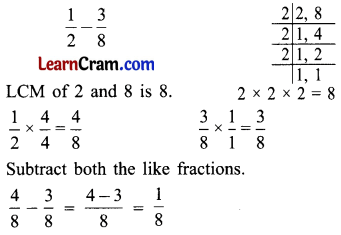(b) $$\frac{9}{10}-\frac{3}{5}$$
Solution: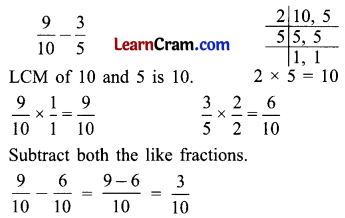(c) $$\frac{11}{12}-\frac{2}{3}$$
Solution: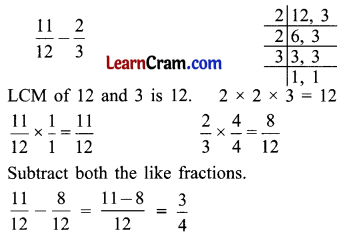(d) $$\frac{2}{3}-\frac{2}{8}$$
Solution: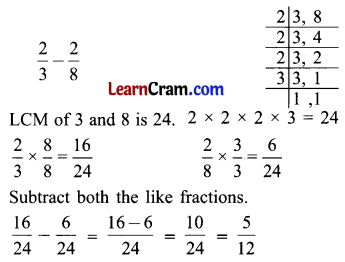(e) $$4 \frac{1}{4}-\frac{3}{8}$$
Solution: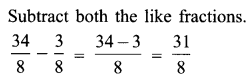(f) $$3 \frac{4}{5}-2 \frac{1}{10}$$
Solution:(g) 4 – $$\frac{3}{8}$$
Solution: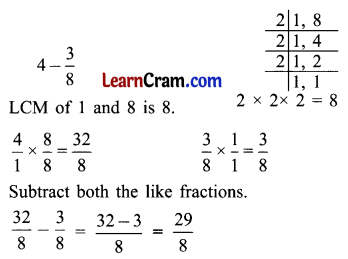(h) $$2 \frac{1}{5}-1 \frac{1}{2}$$
Solution:DAV Class 5 Maths Chapter 4 Worksheet 4 Notes

Example 1.
Add unlike fractions $$\frac{5}{6}+\frac{1}{8}$$
Solution:
LCM of denominators 6 and 8 is 24So, $$\frac{5}{6}+\frac{1}{8}$$ = $$\frac{20}{24}+\frac{3}{24}$$ (Add the numerators, Denominator remains the same)
= $$\frac{23}{24}$$

Example 2.
Subtract $$\frac{7}{5}$$ from $$\frac{23}{10}$$.
Solution:
$$\frac{23}{10}-\frac{7}{5}$$ (Making them like fraction)
LCM of 10 and 5 is 10
= $$\frac{23}{10}-\frac{14}{10}$$
= $$\frac{9}{10}$$ lowest term.Example 3.
Add $$\frac{7}{10}$$, $$\frac{1}{6}$$ and $$\frac{7}{15}$$
Solution:
First convert unlike fractions into like fractions by finding LCM of denominators.
LCM of denominator 10, 6, 15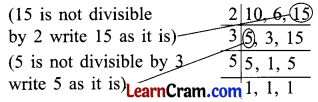Step 1. Write the numbers in a line.
Step 2. Divide the number by common factors.
Step 3. Again divide the number by common factor of one or more numbers.
Step 4. Stop when you get all quotients equal to 1.
$$\frac{7}{10} \times \frac{3}{3}=\frac{21}{30}$$
(30 ÷ 10 = 3)
(Multiply numerator and denominator by 3)

$$\frac{1}{6} \times \frac{5}{5}=\frac{5}{30}$$
(30 ÷ 6 = 5)
(Multiply numerator and denominator by 5)

$$\frac{7}{15} \times \frac{2}{2}=\frac{14}{30}$$
(30 ÷ 15 = 2)
(Multiply numerator and denominator by 2)
= $$\frac{21}{30}+\frac{5}{30}+\frac{14}{30}$$
= $$\frac{21+5+14}{30}$$
= $$\frac{40}{30}$$
= $$\frac{4}{3}$$ (Lowest term)